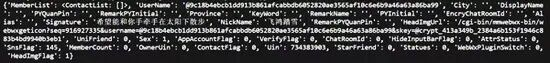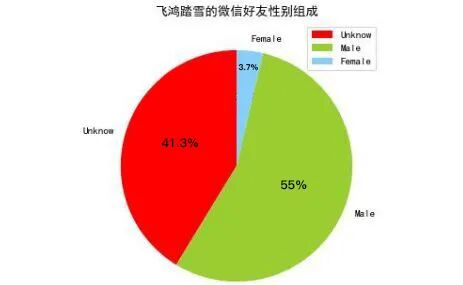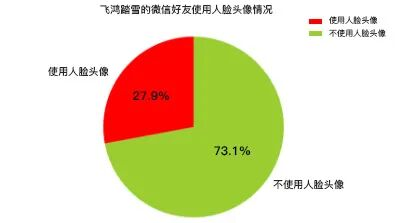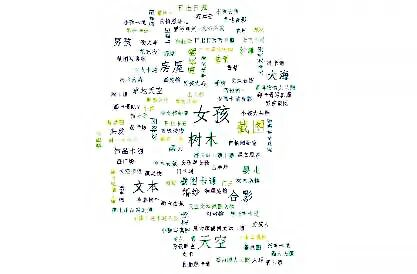# 用Python爬了我的微信好友，他们是这样的…

• itchat：微信网页版接口封装Python版本，在本文中用以获取微信好友信息。
• * jieba：结巴分词的 Python 版本，在本文中用以对文本信息进行分词处理。
• * matplotlib：Python 中图表绘制模块，在本文中用以绘制柱形图和饼图
• * snownlp：一个 Python 中的中文分词模块，在本文中用以对文本信息进行情感判断。
• * PIL：Python 中的图像处理模块，在本文中用以对图片进行处理。
• * numpy：Python中 的数值计算模块，在本文中配合 wordcloud 模块使用。
• * wordcloud：Python 中的词云模块，在本文中用以绘制词云图片。
• * TencentYoutuyun：腾讯优图提供的 Python 版本 SDK ，在本文中用以识别人脸及提取图片标签信息。

01

`itchat.auto_login(hotReload = True) ``friends = itchat.get_friends(update = True)`02

`def analyseSex(firends):`` sexs = list(map(lambda x:x['Sex'],friends[1:])) `` counts = list(map(lambda x:x,Counter(sexs).items())) `` labels = ['Unknow','Male','Female'] `` colors = ['red','yellowgreen','lightskyblue'] `` plt.figure(figsize=(8,5), dpi=80) `` plt.axes(aspect=1) `` plt.pie(counts, #性别统计结果 `` labels=labels, #性别展示标签 `` colors=colors, #饼图区域配色 `` labeldistance = 1.1, #标签距离圆点距离 `` autopct = '%3.1f%%', #饼图区域文本格式 `` shadow = False, #饼图是否显示阴影 `` startangle = 90, #饼图起始角度 `` pctdistance = 0.6 #饼图区域文本距离圆点距离 `` ) `` plt.legend(loc='upper right',) `` plt.title(u'%s的微信好友性别组成' % friends['NickName']) `` plt.show()`03

`def analyseHeadImage(frineds):``# Init Path `` basePath = os.path.abspath('.') `` baseFolder = basePath + '\HeadImages\'``if(os.path.exists(baseFolder) == False): `` os.makedirs(baseFolder) ``# Analyse Images `` faceApi = FaceAPI() `` use_face = 0`` not_use_face = 0`` image_tags = ''``for index in range(1,len(friends)): `` friend = friends[index] ``# Save HeadImages `` imgFile = baseFolder + '\Image%s.jpg' % str(index) `` imgData = itchat.get_head_img(userName = friend['UserName']) ``if(os.path.exists(imgFile) == False): ``with open(imgFile,'wb') as file: `` file.write(imgData) ``# Detect Faces `` time.sleep(1) `` result = faceApi.detectFace(imgFile) ``if result == True: `` use_face += 1``else: `` not_use_face += 1``# Extract Tags `` result = faceApi.extractTags(imgFile) `` image_tags += ','.join(list(map(lambda x:x['tag_name'],result))) `` labels = [u'使用人脸头像',u'不使用人脸头像'] `` counts = [use_face,not_use_face] `` colors = ['red','yellowgreen','lightskyblue'] `` plt.figure(figsize=(8,5), dpi=80) `` plt.axes(aspect=1) `` plt.pie(counts, #性别统计结果 `` labels=labels, #性别展示标签 `` colors=colors, #饼图区域配色 `` labeldistance = 1.1, #标签距离圆点距离 `` autopct = '%3.1f%%', #饼图区域文本格式 `` shadow = False, #饼图是否显示阴影 `` startangle = 90, #饼图起始角度 `` pctdistance = 0.6 #饼图区域文本距离圆点距离 `` ) `` plt.legend(loc='upper right',) `` plt.title(u'%s的微信好友使用人脸头像情况' % friends['NickName']) `` plt.show() `` image_tags = image_tags.encode('iso8859-1').decode('utf-8') `` back_coloring = np.array(Image.open('face.jpg')) `` wordcloud = WordCloud( `` font_path='simfang.ttf', `` background_color="white", `` max_words=1200, `` mask=back_coloring, `` max_font_size=75, `` random_state=45, `` width=800, `` height=480, `` margin=15`` ) `` wordcloud.generate(image_tags) `` plt.imshow(wordcloud) `` plt.axis("off") `` plt.show()`04

`def analyseSignature(friends):`` signatures = ''`` emotions = [] `` pattern = re.compile("1fd.+") ``for friend in friends: `` signature = friend['Signature'] ``if(signature != None): `` signature = signature.strip().replace('span', '').replace('class', '').replace('emoji', '') `` signature = re.sub(r'1f(d.+)','',signature) ``if(len(signature)>0): `` nlp = SnowNLP(signature) `` emotions.append(nlp.sentiments) `` signatures += ' '.join(jieba.analyse.extract_tags(signature,5)) ``with open('signatures.txt','wt',encoding='utf-8') as file: `` file.write(signatures) ``# Sinature WordCloud `` back_coloring = np.array(Image.open('flower.jpg')) `` wordcloud = WordCloud( `` font_path='simfang.ttf', `` background_color="white", `` max_words=1200, `` mask=back_coloring, `` max_font_size=75, `` random_state=45, `` width=960, `` height=720, `` margin=15`` ) `` wordcloud.generate(signatures) `` plt.imshow(wordcloud) `` plt.axis("off") `` plt.show() `` wordcloud.to_file('signatures.jpg') ``# Signature Emotional Judgment `` count_good = len(list(filter(lambda x:x>0.66,emotions))) `` count_normal = len(list(filter(lambda x:x>=0.33 and x<=0.66,emotions))) `` count_bad = len(list(filter(lambda x:x<0.33,emotions))) `` labels = [u'负面消极',u'中性',u'正面积极'] `` values = (count_bad,count_normal,count_good) `` plt.rcParams['font.sans-serif'] = ['simHei'] `` plt.rcParams['axes.unicode_minus'] = False`` plt.xlabel(u'情感判断') `` plt.ylabel(u'频数') `` plt.xticks(range(3),labels) `` plt.legend(loc='upper right',) `` plt.bar(range(3), values, color = 'rgb') `` plt.title(u'%s的微信好友签名信息情感分析' % friends['NickName']) `` plt.show()`

05

`def analyseLocation(friends): `` headers = ['NickName','Province','City'] ``with open('location.csv','w',encoding='utf-8',newline='',) as csvFile: `` writer = csv.DictWriter(csvFile, headers) `` writer.writeheader() ``for friend in friends[1:]: ``row = {} ``row['NickName'] = friend['NickName'] ``row['Province'] = friend['Province'] ``row['City'] = friend['City'] `` writer.writerow(row)`

06